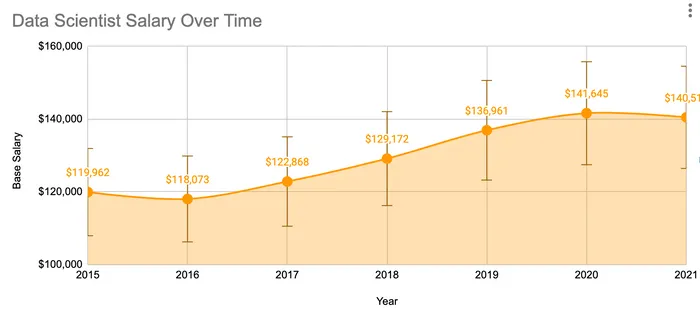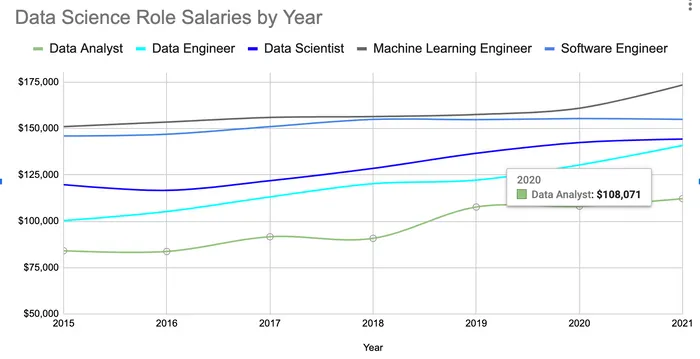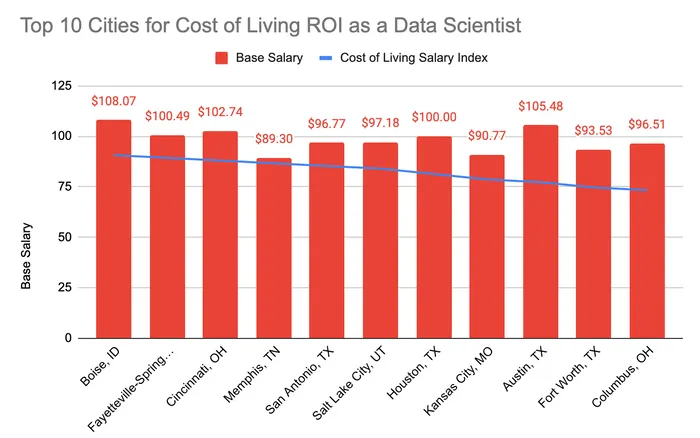# Data Scientist Salaries (Updated for 2022)

Let's dive into data science salaries and see how they vary by seniority, location, and across different companies.

We analyzed over 50,000 data science salaries.

There is base salary, which is a standard everywhere, but with the advent of stock options, restricted stock units (RSUs), annual bonuses, sign on bonuses, 401K matching, and an extremely tight labor market, data science salary numbers can be complex.

$122,831 Average Base Salary$178,864

Average Total Compensation

Min: $70K Max:$183K
Base Salary
Median: $120K Mean (Average):$123K
Data points: 29,965
Min: $19K Max:$389K
Total Compensation
Median: $162K Mean (Average):$179K
Data points: 4,076

## Data Scientist Salaries Over Time

We analyzed data going all the way back to 2015 and realized that salaries for data scientists have gone up as the field as grown.

Because the average across all time periods isn’t really that helpful, we tried to add a recency weighted scale that would adjust the salaries for the more recent additions. As you can see, in the last two years, the average data scientist salary is actually around $140K.We initially used a linear recency scale - but as you can see it’s still averaged to pre-2018. So in the future we might us an exponential one to really validate our findings and show the most up to date accurate information. ## Data Scientist Salary Compared to other Positions Data science is a term to describe many different positions across a spectrum of roles. If we compare the salary of positions named “Data Scientist” across data engineers and machine learning engineers while also looking at other tech jobs like product managers and software engineers, data scientists fall neatly in the upper levels, but the variance is pretty high.$85K
$235K Product Manager Median:$155K
Mean (Average): $158K Data points: 7,597$80K
$225K Machine Learning Engineer Median:$147K
Mean (Average): $149K Data points: 4,290$70K
$183K Data Scientist Median:$120K
Mean (Average): $123K Data points: 29,965$61K
$196K Software Engineer Median:$105K
Mean (Average): $116K Data points: 278,458$64K
$168K Data Engineer Median:$100K
Mean (Average): $107K Data points: 28,370$56K
$150K Product Analyst Median:$90K
Mean (Average): $95K Data points: 870$56K
$141K Business Intelligence Median:$90K
Mean (Average): $94K Data points: 10,675$49K
$174K Research Scientist Median:$80K
Mean (Average): $94K Data points: 14,454$46K
$145K Data Analyst Median:$75K
Mean (Average): $83K Data points: 32,107$44K
$160K Growth Marketing Analyst Median:$68K
Mean (Average): $83K Data points: 1,919$55K
$121K Business Analyst Median:$76K
Mean (Average): $81K Data points: 49,298 Most data science positions fall under different position titles depending on the actual role. From the graph we can see that on average the Product Manager role pays the most with a$158,061 base salary while the Business Analyst role on average pays the least with a $80,732 base salary. Data engineers and data analysts salaries have also increased by 35% over the past 5 years compared to a 6% increase by software engineers. But most data science roles are just playing catch up. Data engineers and data scientists made on average around $140K in base pay in 2021 compared to$155K for software engineers.Machine learning engineers were still the highest paying out of all data positions, averaging$175K in base salary in 2021.

## Data Scientist Salary by Seniority Levels

It’s undeniable that years of experience is by far the biggest factor to influence salaries.

While most titles don’t have seniority titles, we scraped data and combined seniority titles with years of experience to create buckets representative of how we would envision a regular career path forming:

• Individual contributor route → Entry level, Mid-level, Senior, Principal, Staff, Architect, etc…

• Manager route → Entry level, Mid-level, Senior, Manager, Director, VP, etc…

Here we’ve bucketed almost all individuals with 5+ years of experience or varying levels of senior individual contributor titles (staff, principal, etc…) into one bucket called “Senior Data Scientists”. This drastically reduces the complexity around understanding how different organizations set their own levels (is L1 or L3 the bottom?) and titles (is principal more senior than staff?).

Seniority Title Seniority Categorization
Entry Level Data Scientist 0-1 years of relevant experience
Mid-Level Data Scientist 2-5 years of relevant experience
Senior Data Scientist 6+ years of relevant experience
Data Science Manager Manager in job title
Director or VP of Data Science Director or higher in job title
$115K$304K
Executive
Median: $190K Mean (Average):$191K
Data points: 13
$77K$220K
Manager
Median: $138K Mean (Average):$142K
Data points: 822
$84K$210K
Senior
Median: $140K Mean (Average):$144K
Data points: 4,376
$77K$180K
Mid-Level
Median: $138K Mean (Average):$133K
Data points: 4,128
$60K$155K
Entry Level
Median: $90K Mean (Average):$100K
Data points: 830

Seniority can increase the pay of a Data Scientist. Here are the base salaries of a Data Scientist grouped into 5 seniority categories.

We’re seeing that on average, once you have a few years of experience under your belt, the base salary and total compensation numbers skyrocket.

So just note that after one or two years at a company as an entry level data scientist, and you’re not getting a significant raise, it’s definitely in your best interest to try to move jobs.

Additionally the data suggests that many senior data scientists on average make more in total compensation than data science managers.

This is especially due to the number of senior data scientists on staff at larger FAANG companies where an individual contributor with 10+ years of experience can make tons of money given their leverage in increasing company revenue.

## Top Cities for Data Scientist Salaries

Most data science salaries vary a lot by location. But Covid-19 and the shift to remote work really changed how we value location based salaries.

For example here are the cities with the highest base salaries. And it’s no surprise that average base data science salaries in San Francisco and Seattle come in on top with at over $145K and$130K, respectively.

$92K$200K
San Francisco, CA
Median: $142K Mean (Average):$144K
Data points: 8,901
$96K$228K
Sydney, Australia
Median: $110K Mean (Average):$140K
Data points: 5
$95K$185K
Zurich, Switzerland
Median: $137K Mean (Average):$136K
Data points: 7
$97K$175K
Tel Aviv, Israel
Median: $133K Mean (Average):$136K
Data points: 13
$91K$179K
Seattle, WA
Median: $135K Mean (Average):$135K
Data points: 4,817
$78K$168K
Charleston, SC
Median: $144K Mean (Average):$128K
Data points: 5
$70K$180K
Los Angeles, CA
Median: $119K Mean (Average):$126K
Data points: 885
$73K$182K
New York, NY
Median: $120K Mean (Average):$123K
Data points: 3,336
$70K$157K
Median: $133K Mean (Average):$121K
Data points: 6
$59K$210K
Omaha, NE
Median: $120K Mean (Average):$120K
Data points: 57
$76K$187K
Memphis, TN
Median: $102K Mean (Average):$118K
Data points: 70
$86K$154K
Dublin, Ireland
Median: $114K Mean (Average):$117K
Data points: 8
$67K$170K
Baltimore, MD
Median: $111K Mean (Average):$116K
Data points: 63
$73K$160K
San Diego, CA
Median: $110K Mean (Average):$115K
Data points: 249
$71K$160K
Boston, MA
Median: $111K Mean (Average):$115K
Data points: 1,608

The Data Scientist salary in Geneva, Switzerland is the highest paying salary with a $183,000 average base salary. The Data Scientist salary in Warsaw, Poland is the lowest paying salary with$45,000 average base salary.

But with the advent of remote work, employers started making choices whether to normalize salaries across the entire U.S. or based on where you were living. So if we look at the best cities to live, normalized data science salaries in Austin, Boise, Arkansas, and Cincinnati are among the best.## How Education Level Affects Data Science Salaries

Education has a clear affect on how much you can earn as a data scientist. A master’s degree in a data science-related field, for example, typically results in a $10,000-$15,000 increase in base salary.

### Master’s Degree Salaries

A master’s degree is increasingly a preferred qualification for tech jobs at FAANG companies. The most common fields of study for data science include data analytics, data science, statistics and machine learning. Here are some of the top master’s in data science salaries:

• Data Science Master’s - $137,000 / base salary - **[Business Analytics Master's](https://www.interviewquery.com/p/masters-in-business-analytics-salary)** -$143,000 / base salary
• Data Analytics Master’s - $90,000 / base salary - **[Health Informatics Master's](https://www.interviewquery.com/p/masters-in-health-informatics-salary)** -$88,000 / base salary

### PhD Salaries

PhD are a preferred qualification for a number of data science specialized research roles. PhD programs in mathematics, statistics, machine learning and data science are all options to land research jobs in tech, finance and more. Here are common salaries by degree obtained:

• Statistics PhD - $148,000 / base salary - **[Mathematics PhD](https://www.interviewquery.com/p/phd-in-mathematics-salary) -**$148,000 / base salary
• Economics PhD - $150,000 / base salary ## Top Companies for Data Scientist Salaries Lastly analyzing the top companies that hire for data scientists, we can see the FAANG companies all around the top.$112K
$649K Netflix Median:$303K
Mean (Average): $319K Data points: 86$113K
$284K Roblox Median:$223K
Mean (Average): $216K Data points: 22$106K
$260K Stitch Fix Median:$223K
Mean (Average): $200K Data points: 72$154K
$264K Citadel Llc Median:$190K
Mean (Average): $198K Data points: 9$153K
$218K Reddit, Inc. Median:$210K
Mean (Average): $190K Data points: 13$181K
$198K Albertsons Median:$190K
Mean (Average): $189K Data points: 5$90K
$340K Goldman Sachs Median:$175K
Mean (Average): $187K Data points: 12$144K
$230K Wish - Shopping Made Fun! Median:$190K
Mean (Average): $187K Data points: 8$137K
$224K Roku Inc. Median:$189K
Mean (Average): $182K Data points: 5$100K
$233K Bytedance Inc. Median:$177K
Mean (Average): $178K Data points: 63$128K
$242K Tiktok Median:$180K
Mean (Average): $178K Data points: 16$144K
$237K Slack Median:$158K
Mean (Average): $177K Data points: 5$130K
$224K Airbnb Median:$175K
Mean (Average): $174K Data points: 182$73K
$240K Twilio Inc. Median:$188K
Mean (Average): $174K Data points: 6$147K
$200K Datarobot Median:$173K
Mean (Average): $173K Data points: 14 The Data Scientist at Netflix salary is the highest paying salary with a$318,757 average base salary. The Data Scientist at Gojek tech salary is the lowest paying salary with \$41,000 average base salary.